GEOMETRY 1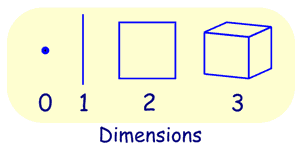## Point, Line, Plane and Solid

A Point has no dimensions, only position
A Line is one-dimensional
A Plane is two dimensional (2D)
A Solid is three-dimensional (3D)

Acute angle:

An angle whose measure is less than 90 degrees. The following is an acute angle.Right angle:

An angle whose measure is 90 degrees. The following is a right angle.Obtuse angle:

An angle whose measure is bigger than 90 degrees but less than 180 degrees. Thus, it is between 90 degrees and 180 degrees. The following is an obtuse angle.Straight angle

An angle whose measure is 180 degrees.Thus, a straight angle look like a straight line. The following is a straight angle.Reflex angle:

An angle whose measure is bigger than 180 degrees but less than 360 degrees.The following is a reflex angle.Angle with a common vertex and one common side. <1 and <2, are adjacent angles.Complementary angles:

Two angles whose measures add to 90 degrees. Angle 1 and angle 2 are complementary angles because together they form a right angle.

Note that angle 1 and angle 2 do not have to be adjacent to be complementary as long as they add up to 90 degreesThese two angles (40° and 50°) areComplementary Angles, because they add up to 90°.Notice that together they make a right angle.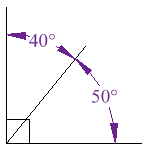But the angles don't have to be together.These two are complementary because 27° + 63° = 90°Supplementary angles:

Two angles whose measures add to 180 degrees. The following are supplementary angles.# Supplementary Angles

Two Angles are Supplementary if they add up to 180 degrees.

 These two angles (140° and 40°) are Supplementary Angles, because they add up to 180°.Notice that together they make a straight angle.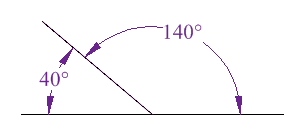But the angles don't have to be together.These two are supplementary because 60° + 120° = 180°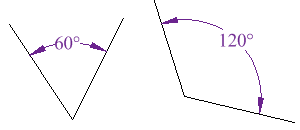Vertical angles:

Angles that have a common vertex and whose sides are formed by the same lines. The following(angle 1 and angle 2) are vertical angles.When two parallel lines are crossed by a third line(Transversal), 8 angles are formed. Take a look at the following figureAngles 3,4,5,8 are interior angles

Angles 1,2,6,7 are exterior angles

Alternate interior angles:

Pairs of interior angles on opposite sides of the transversal.

For instance, angle 3 and angle 5 are alternate interior angles. Angle 4 and angle 8 are also alternate interior angles.

Alternate exterior angles:

Pairs of exterior angles on opposite sides of the transversal.

Angle 2 and angle 7 are alternate exterior angles.

Corresponding angles:

Pairs of angles that are in similar positions.

Angle 3 and angle 2 are corresponding angles.

Angle 5 and angle 7 are corresponding angles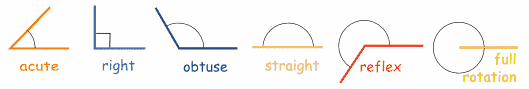Reflexive Property A quantity is congruent (equal) to itself.  a = a Symmetric Property If a = b, then b = a. Transitive Property If a = b and b = c, then a = c. Addition Postulate If equal quantities are added to equal quantities, the sums are equal. Subtraction Postulate If equal quantities are subtracted from equal quantities, the differences are equal. Multiplication Postulate If equal quantities are multiplied by equal quantities, the products are equal.  (also Doubles of equal quantities are equal.) Division Postulate If equal quantities are divided by equal nonzero quantities, the quotients are equal. (also Halves of equal quantities are equal.) Substitution Postulate A quantity may be substituted for its equal in any expression. Partition Postulate The whole is equal to the sum of its parts.Also:  Betweeness of Points:  AB + BC = ACAngle Addition Postulate:  m Construction Two points determine a straight line. Construction From a given point on (or not on) a line, one and only one perpendicular can be drawn to the line.

Angles:

 Right Angles All right angles are congruent. Straight Angles All straight angles are congruent. Congruent Supplements Supplements of the same angle, or congruent angles, are congruent. Congruent Complements Complements of the same angle, or congruent angles, are congruent. Linear Pair If two angles form a linear pair, they are supplementary. Vertical Angles Vertical angles are congruent. Triangle Sum The sum of the interior angles of a triangle is 180º. Exterior Angle The measure of an exterior angle of a triangle is equal to the sum of the measures of the two non-adjacent interior angles.The measure of an exterior angle of a triangle is greater than either non-adjacent interior angle. Base Angle Theorem(Isosceles Triangle) If two sides of a triangle are congruent, the angles opposite these sides are congruent. Base Angle Converse(Isosceles Triangle) If two angles of a triangle are congruent, the sides opposite these angles are congruent.

Triangles:

 Side-Side-Side (SSS) Congruence If three sides of one triangle are congruent to three sides of  another triangle, then the triangles are congruent. Side-Angle-Side (SAS) Congruence If two sides and the included angle of one triangle are congruent to the corresponding parts of another triangle, the triangles are congruent. Angle-Side-Angle (ASA) Congruence If two angles and the included side of one triangle are congruent to the corresponding parts of another triangle, the triangles are congruent. Angle-Angle-Side (AAS) Congruence If two angles and the non-included side of one triangle are congruent to the corresponding parts of another triangle, the triangles are congruent. Hypotenuse-Leg (HL) Congruence (right triangle) If the hypotenuse and leg of one right triangle are congruent to the corresponding parts of another right triangle, the two right triangles are congruent. CPCTC Corresponding parts of congruent triangles are congruent. Angle-Angle (AA) Similarity If two angles of one triangle are congruent to two angles of another triangle, the triangles are similar. SSS for Similarity If the three sets of corresponding sides of two triangles are in proportion, the triangles are similar. SAS for Similarity If an angle of one triangle is congruent to the corresponding angle of another triangle and the lengths of the sides including these angles are in proportion, the triangles are similar. Side Proportionality If two triangles are similar, the corresponding sides are in proportion. Mid-segment Theorem(also called mid-line) The segment connecting the midpoints of two sides of a triangle is parallel to the third side and is half as long. Sum of Two Sides The sum of the lengths of any two sides of a triangle must be greater than the third side Longest Side In a triangle, the longest side is across from the largest angle.In a triangle, the largest angle is across from the longest side. Altitude Rule The altitude to the hypotenuse of a right triangle is the mean proportional between the segments into which it divides the hypotenuse. Leg Rule Each leg of a right triangle is the mean proportional between the hypotenuse and the projection of the leg on the hypotenuse.

Parallels:

 Corresponding Angles If two parallel lines are cut by a transversal, then the pairs of corresponding angles are congruent. Corresponding Angles Converse If two lines are cut by a transversal and the corresponding angles are congruent, the lines are parallel. Alternate Interior Angles If two parallel lines are cut by a transversal, then the alternate interior angles are congruent. Alternate Exterior Angles If two parallel lines are cut by a transversal, then the alternate exterior angles are congruent. Interiors on Same Side If two parallel lines are cut by a transversal, the interior angles on the same side of the transversal are supplementary. Alternate Interior Angles Converse If two lines are cut by a transversal and the alternate interior angles are congruent, the lines are parallel. Alternate Exterior AnglesConverse If two lines are cut by a transversal and the alternate exterior angles are congruent, the lines are parallel. Interiors on Same Side Converse If two lines are cut by a transversal and the interior angles on the same side of the transversal are supplementary, the lines are parallel.